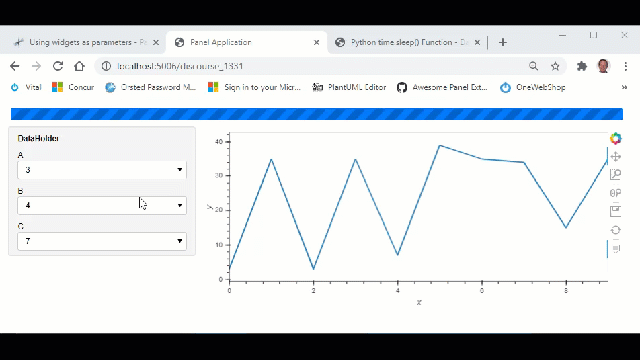# Using parameters like widgets

Hi

I am trying to realize the following panel structure.

• widgets - a, b
• widget - c (selection options change with a or b)
• custom data - “data” (changes with a or b). Expensive step.
• plot 1 - changes with widget c (depends on custom data)
• plot 2 - changes with widget c (depends on custom data)

Current code to do this is:

``````a = pn.widgets.Select(name="a", options=[1, 2, 3])
b = pn.widgets.Select(name="b", options=[4, 5, 6])

c = pn.widgets.Select(name="c", options=)
expensive = {"plot_data": big_df}

@pn.depends(a, b, watch=True)
def _update_expensive(a, b):
print("expensive step")
expensive["plot_data"] = ....

print("update widget c")
c.options = list(range(0, a + b))

@pn.depends(c)
def _update_plot_one(c):
return ...
@pn.depends(c)
def _update_plot_two(c):
return ...
``````

The current code to update the custom data is written along with the routine updating the widget `c`, since they have the same parent dependencies. However, with more intermediate variables, it’ll become unwieldy.

I want to create a custom class instead of a dictionary that can update itself with whenever widgets `a` or `b` change but can’t quite figure out how to specify widgets as dependencies, since they are not regular parameters. Is there a way to do this without writing the entire GUI as a subclass of `param.Parameterized`?

``````class DataHolder(param.Parameterized):
w1 = param.Selector()
w2 = param.Selector()

def __init__(self, widget_a, widget_b):
super().__init__(w1 = widget_a, w2 = widget_b)
self._updater()

@param.depends(w1, w2, watch=True)
def _updater(self):
self.data = ....
``````

Thank you for looking at this.

The documentation points out using the low level `links` to set up callbacks, which offers one way to solve this.

``````class DataHolder:
def __init__(self, widget_a, widget_b):
self.w1 = widget_a
self.w2 = widget_b
self.w1.param.watch(self._updater, 'value')
self.w2.param.watch(self._updater, 'value')
self._updater()

def _updater(self):
self.data = ....
``````

Though I’d like to be able to use this as a dependency for downstream panes/widgets, so changes to this custom datatype trigger updates on them. The desired sequence of update events is:

`widget a/b -> data -> widget c -> plot1/plot2`.

With the current form, both `DataHolder` and the `widget c` receive updates on a change to either `widget a` or `widget b`, and the order in which they get updated isn’t guaranteed.

I would actually not use the widgets as parameters. Instead I would create a parameterized model like the below.``````import param
import panel as pn
import hvplot.pandas
import pandas as pd
from random import randint, randrange
import time

class DataHolder(param.Parameterized):
value_a = param.ObjectSelector(default=1, objects=[1, 2, 3], label="A")
value_b = param.ObjectSelector(default=4, objects=[4, 5, 6], label="B")
value_c = param.ObjectSelector(default=7, objects=[7, 8, 9], label="C")

data = param.DataFrame()
view = param.Parameter()

def __init__(self, **params):
super().__init__(**params)

self._sleep=0

self._create_view()
self._update_data()
self._update_plot()

self._sleep=5

def _create_view(self):
self.settings_panel = pn.Param(self, parameters=["value_a", "value_b", "value_c"])
self.plot_panel = pn.pane.HoloViews(sizing_mode="stretch_width")
self.progress = pn.widgets.Progress(sizing_mode="stretch_width", bar_color="primary")
self.view = pn.Column(
self.progress,
pn.Row(
pn.WidgetBox(self.settings_panel), self.plot_panel, sizing_mode="stretch_width"
)
)

@param.depends("value_a", "value_b", watch=True)
def _update_data(self, *event):
self.progress.active = True
time.sleep(self._sleep)
self.data = pd.DataFrame(
{
"x": [i for i in range(0, 10)],
"y": [self.value_a + randint(0,10) * self.value_b for i in range(0, 10)],
}
)
print("data updated")
self.progress.active = False

@param.depends("data", "value_c", watch=True)
def _update_plot(self, *event):
if self.data is None:
return

data = self.data.copy()
data.loc[self.value_c, "y"] = self.value_c + data.loc[self.value_c, "y"]
self.plot_panel.object = data.hvplot(x="x", y="y")
print("plot updated")

DataHolder().view.servable()

``````
1 Like

Thank you Marc for the exhaustive answer! Since installing Panel yesterday, snippets posted by you on the forum have helped me a lot.

When serving the dashboard as an application, it clearly makes sense to put the GUI in a single `param.Parameterized` class, since everything is specified cleanly. Though for working in a jupyter notebook, it seems a bit inconvenient if I only want to add widgets around 3/4 parameters. Working with widgets feels natural in that changes needed are:

• replace variables in my imperative code with `widget.value`.
• add a `panel.depends` decorator around the methods that should be updated.

A panel/param construct that can wrap an arbitrary python variable and also have other widgets/params as parent/child dependencies, would probably be helpful here. I’ll look into subclassing one of the exposed base classes in the `param` library. Have you come across a similar use case?

1 Like

I came up with this but it seems to either throw a javascript error or run the update method multiple times for a single change, so I’ll go with creating the big `Parameterized` class. Thanks @Marc.

``````class FakeWidget(pn.widgets.Widget):
value = param.Parameter(default=None)

def __init__(self, name, value):
super().__init__(name=name, value=value)

data_holder = FakeWidget("data_holder", data)
@pn.depends(data_holder)
def _update_(data_holder):
# update downstream widgets
``````

Would replacing `pn.widgets.Widget` in your code with `param.Parameterized` solve the issues?

No, that didn’t work. I think the `pn.depends` decorator needs widgets to be passed in, so inheriting from a panel Widget seems necessary.
My hunch is a widget also needs some corresponding javascript code (the web console had an error citing an unregistered class), so just subclassing in python didn’t work.

I apologize since you have already given a working example, could you please point me to any examples where the GUI is split between >1 `param.Parameterized` classes, and how to make them depend on one other.